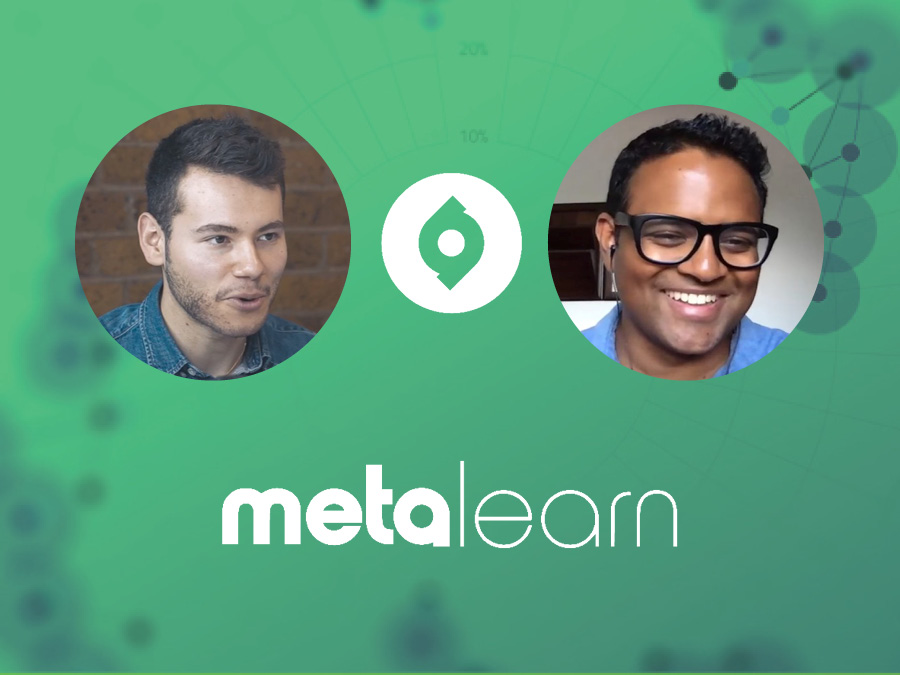Learning

ML102: Equations That Changed The World - Kalid Azad on Newton's Universal Law of Gravity

This episode is a special one on Isaac Newton’s Universal Law of Gravity. Discover Newton’s backstory and how it influenced his work, the mechanics of the equation in a way that you can understand and the implications of the equation for our view of the Universe.This episode is a special one on equations that changed the world.

And what better one to start with than Isaac Newton’s Universal Law of Gravity – an equation that ultimately helped us put a man on the moon!

In simple terms the equation reads:

Earth’s Gravitational Force = (G x M x m) / D squared

Earth’s Force = (Gravitational Constant x Earth’s Mass x Mass of the Object) /  Distance between the Earth and Object Squared

What this equation allowed the smart scientists at NASA to do was to to establish with accuracy where exactly the moon was, which was no mean feat in itself.

And by calculating the rate at which gravity diminished on the way to the moon, they determined what size rocket they’d need and the best route to get there.

Today’s guest, Kalid Azad, is the founder of Better Explained, one of the world's most popular maths websites that makes hard concepts easy to understand.

After studying Computer Science at Princeton, Kalid spent a few years at Microsoft as a program manager, founded a Y Combinator startup, and currently works as a developer.

Kalid uses an intuition first approach to explain difficult ideas in a way that anyone can understand and this makes him the perfect guide.

In this episode we discuss:

- Newton’s backstory and how it influenced his work
- The mechanics of the equation in a way that you can understand
- The implications of the equation for our view of the Universe

So whether you’re looking to find out more about Newton, dive deeper into the equation and its importance, or explore maths and physics in a way that’s fun and accessible, this episode has you covered.

# MASTER YOUR LEARNINGWITH OUR FREE STARTER PACK

- 5x Video Lessons on the 5 key MetaLearning Principles
- The Learning Toolkit featuring my top 10 Learning Apps

Enter Your Details Below for Instant Access
Oops! Something went wrong while submitting the form
100% privacy. No Spam. Ever.
CLOSE